# Community

Blog Events Webinars Tutorials Forum
×
Community Blog Alibaba Cloud Machine Learning Platform for AI: Heart Disease Prediction

# Alibaba Cloud Machine Learning Platform for AI: Heart Disease Prediction

This article will illustrate how to build a heart disease prediction case through the Alibaba Cloud Machine Learning Platform for Artificial Intelligence using real data.

By Garvin Li

Heart disease is one of the leading cause of death worldwide; approximately one third of the world's deaths are caused by heart disease. In China, hundreds of thousands of people die of heart disease every year. Researchers from across the globe are actively finding ways to prevent and accurately diagnose heart-related diseases at an early stage. One of the common approaches to do this is by analyzing historical medical data using big data and machine learning technologies.

If we can analyze the impact of different features on heart disease through data mining by extracting physical examination indicators, we can predict and ideally prevent of heart diseases altogether. This article will illustrate how to build a heart disease prediction case through the Alibaba Cloud Machine Learning Platform for Artificial Intelligence (PAI) using real data.

## Dataset Introduction

The data source is UCI open source dataset heart_disease. It contains physical data from 303 patients with heart disease in a certain area of the United States. The detailed fields are as follows: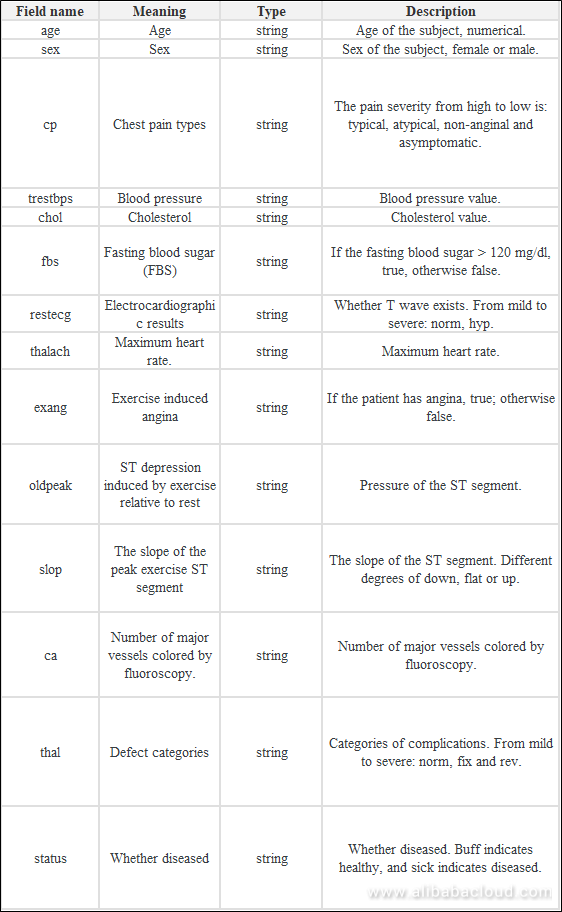## Data Exploration Procedure

The data mining process is as follows: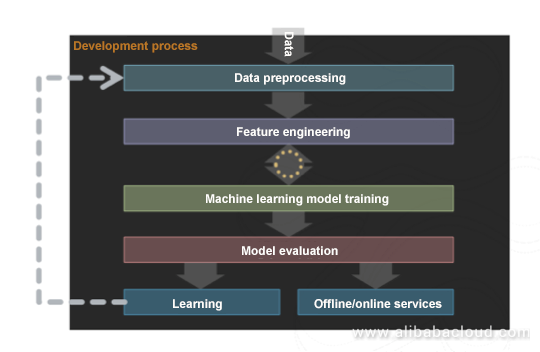Overall experience procedure: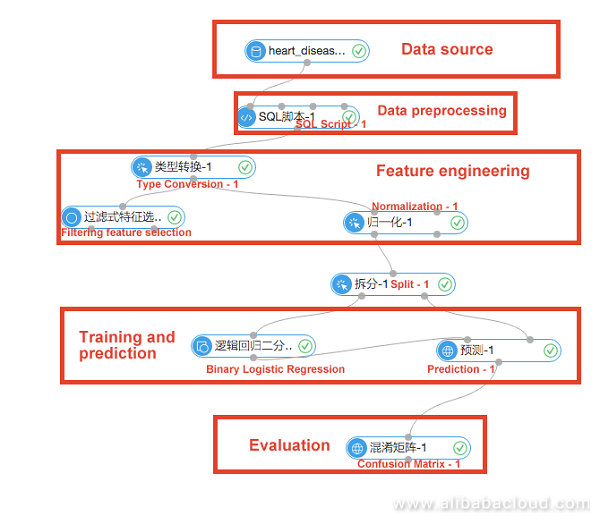## 1. Data Preprocessing

Data preprocessing is also called data cleansing and mainly serves for data de-noising, filling missing values and type conversion operations before the data enters the algorithm procedure. The input data for this experiment consisted of 14 features and 1 target column. The problem to be addressed is to predict whether the user will suffer heart disease based on the user's physical indicators. Each sample is either suffered or not.

This classification experiment adopts the linear model logistic regression; the required input features are all double-type data, as shown in the figure below.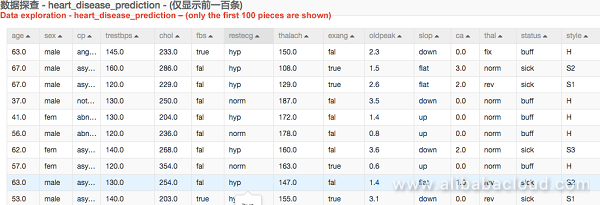A lot of data in the figure above is text description. During data preprocessing, we need to convert strings into numerals based on the meaning of each field.

Boolean data

For example, the field "sex" has two forms: female and male, which can be presented as 0 and 1 respectively.

Multi-value data

For example, the field "cp", which indicates chest pain. We can map the severity from low to high into numerical values of 0 to 3.

Data preprocessing is implemented through SQL scripts.

``````1.
2.    select age,
3.    (case sex when 'male' then 1 else 0 end) as sex,
4.    (case cp when 'angina' then 0  when 'notang' then 1 else 2 end) as cp,
5.    trestbps,
6.    chol,
7.    (case fbs when 'true' then 1 else 0 end) as fbs,
8.    (case restecg when 'norm' then 0  when 'abn' then 1 else 2 end) as restecg,
9.    thalach,
10.    (case exang when 'true' then 1 else 0 end) as exang,
11.    oldpeak,
12.    (case slop when 'up' then 0  when 'flat' then 1 else 2 end) as slop,
13.    ca,
14.    (case thal when 'norm' then 0  when 'fix' then 1 else 2 end) as thal,
15.    (case status  when 'sick' then 1 else 0 end) as ifHealth
16.    from  \${t1};``````

## 2. Feature Engineering

The feature engineering mainly includes feature derivation and scale variation. In this example, there are two components for feature engineering.

Filtering feature selection

Determine the impact of each feature on the results and express with entropy and Gini coefficient. Right click the component and select View Evaluation Report to display the final result, as shown in the following figure.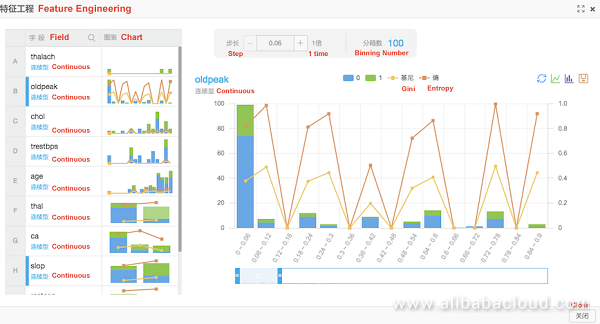Normalization

Change the range of values for each feature to between 0 and 1, which removes the effect of the dimension on the result. The equation is: result = (val-min) / (max - min). This experiment uses binary logistic regression for model training and needs to remove the dimensional impact for each feature. The normalization results are shown in the figure below.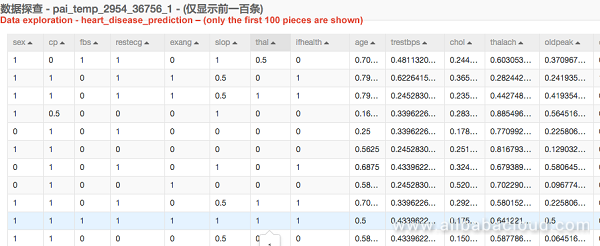## 3. Model Training and Prediction

Supervised learning uses training models with known results. Since whether each sample has heart disease is known, this experiment is classified as supervised learning. The problem to be addressed is to predict whether a group of users suffer from heart disease.

Splitting

First, the data is divided into two parts by component splitting. This experiment split the data based on a ratio of 7:3 for the training set and the prediction set. The training set data flows into the binary logistic regression component for model training. The prediction set data flows into the prediction component.

Binary logistic regression

Logistic regression is a linear model where classification is achieved by computing the threshold value of the results (see relevant documentation for detailed algorithm). Ready models after logical regression can be viewed in the model tab.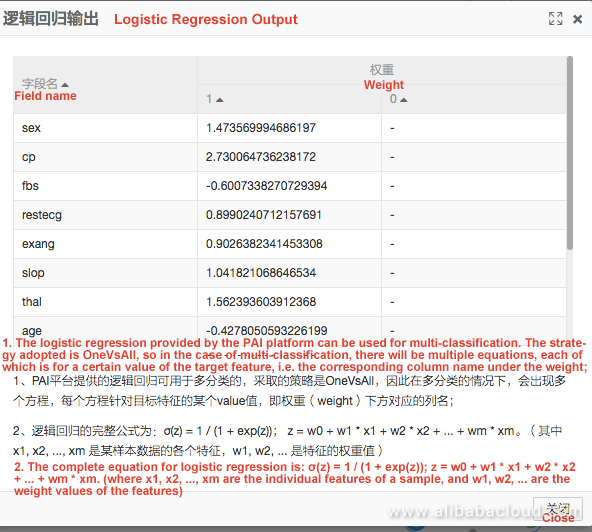Prediction

The two inputs of the prediction component are the model and the prediction set respectively. The prediction result shows the predicted data, real data and the probability of different results in each group.

## 4. Evaluation

Parameters such as the accuracy of the model can be viewed through the confusion matrix component.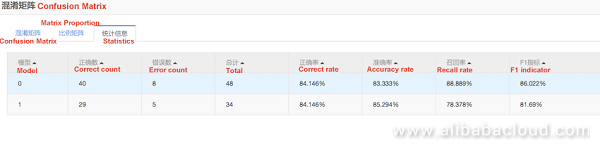This component makes it easy to evaluate models based on the accuracy of the predictions.

## Summary

From the above data exploration procedures we can draw the following conclusions.

Model weight

Through the weights of the corresponding features of each model, the impact of the features on the results can be roughly analyzed. If the model weights are as follows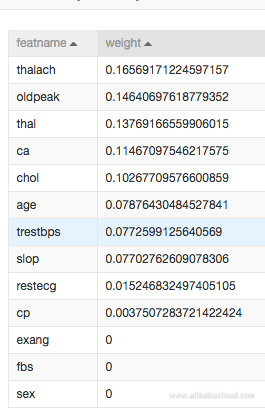The thalach (maximum heart rate achieved) generates the biggest impact on whether or not heart disease occurs.

Sex has no impact on whether or not heart disease occurs.

Model effect

The 14 features provided in this article can help to achieve a heart disease prediction accuracy of more than 80%. The model can be used for prediction to assist physicians in the prevention and treatment of heart disease.

To learn more about Alibaba Cloud Machine Learning Platform for Artificial Intelligence (PAI), visit www.alibabacloud.com/product/machine-learning

0 0 0
Share on

# Related Products

• ##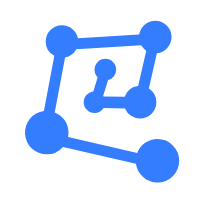Machine Learning Platform For AI

An end-to-end platform that provides various machine learning algorithms to meet your data mining and analysis requirements.

• ##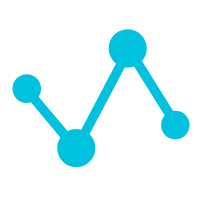Machine Translation

Relying on Alibaba's leading natural language processing and deep learning technology.

• ##Data Transport

A secure solution to migrate TB-level or PB-level data to Alibaba Cloud.

• ##Data Lake Analytics

A premium, serverless, and interactive analytics service